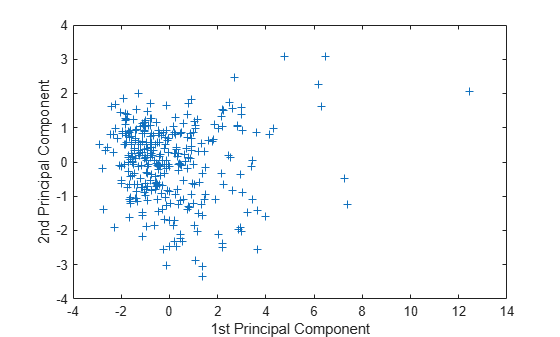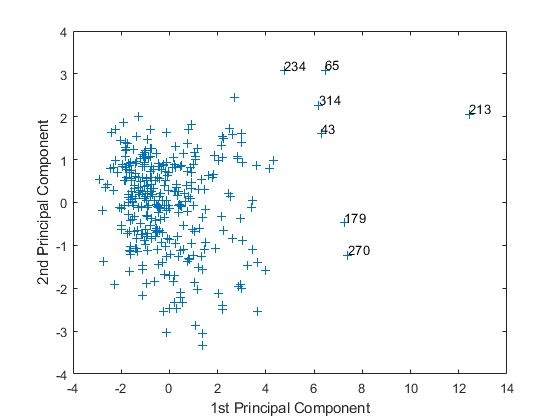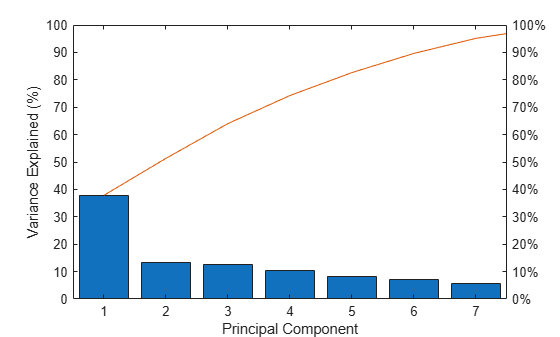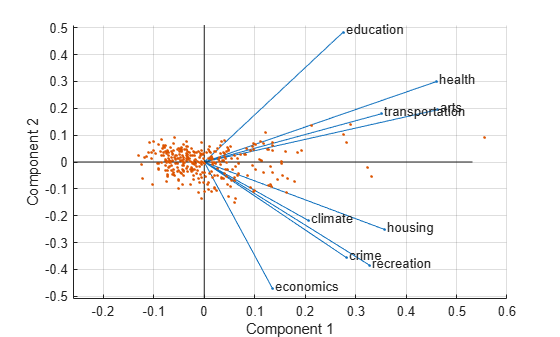# Analyze Quality of Life in U.S. Cities Using PCA

This example shows how to perform a weighted principal components analysis and interpret the results.

Load the sample data. The data includes ratings for 9 different indicators of the quality of life in 329 U.S. cities. These are climate, housing, health, crime, transportation, education, arts, recreation, and economics. For each category, a higher rating is better. For example, a higher rating for crime means a lower crime rate.

Display the `categories` variable.

```load cities categories```
```categories = 9x14 char array 'climate ' 'housing ' 'health ' 'crime ' 'transportation' 'education ' 'arts ' 'recreation ' 'economics ' ```

In total, the `cities` data set contains three variables:

• `categories`, a character matrix containing the names of the indices

• `names`, a character matrix containing the 329 city names

• `ratings`, the data matrix with 329 rows and 9 columns

### Plot Data

Make a box plot to look at the distribution of the `ratings` data.

```figure() boxplot(ratings,'Orientation','horizontal','Labels',categories)```There is more variability in the ratings of the arts and housing than in the ratings of crime and climate.

### Check Pairwise Correlation

Check the pairwise correlation between the variables.

`C = corr(ratings,ratings);`

The correlation among some variables is as high as 0.85. Principal components analysis constructs independent new variables which are linear combinations of the original variables.

### Compute Principal Components

When all variables are in the same unit, it is appropriate to compute principal components for raw data. When the variables are in different units or the difference in the variance of different columns is substantial (as in this case), scaling of the data or use of weights is often preferable.

Perform the principal component analysis by using the inverse variances of the ratings as weights.

```w = 1./var(ratings); [wcoeff,score,latent,tsquared,explained] = pca(ratings, ... 'VariableWeights',w);```

Or equivalently:

```[wcoeff,score,latent,tsquared,explained] = pca(ratings, ... 'VariableWeights','variance'); ```

The following sections explain the five outputs of `pca`.

### Component Coefficients

The first output `wcoeff` contains the coefficients of the principal components.

The first three principal component coefficient vectors are:

`c3 = wcoeff(:,1:3)`
```c3 = 9×3 103 × 0.0249 -0.0263 -0.0834 0.8504 -0.5978 -0.4965 0.4616 0.3004 -0.0073 0.1005 -0.1269 0.0661 0.5096 0.2606 0.2124 0.0883 0.1551 0.0737 2.1496 0.9043 -0.1229 0.2649 -0.3106 -0.0411 0.1469 -0.5111 0.6586 ```

These coefficients are weighted, hence the coefficient matrix is not orthonormal.

### Transform Coefficients

Transform the coefficients so that they are orthonormal.

`coefforth = diag(std(ratings))\wcoeff;`

Note that if you use a weights vector, `w`, while conducting the `pca`, then

```coefforth = diag(sqrt(w))*wcoeff; ```

### Check Coefficients

The transformed coefficients are now orthonormal.

```I = coefforth'*coefforth; I(1:3,1:3)```
```ans = 3×3 1.0000 0.0000 0.0000 0.0000 1.0000 -0.0000 0.0000 -0.0000 1.0000 ```

### Component Scores

The second output `score` contains the coordinates of the original data in the new coordinate system defined by the principal components. The `score` matrix is the same size as the input data matrix. You can also obtain the component scores using the orthonormal coefficients and the standardized ratings as follows.

`cscores = zscore(ratings)*coefforth;`

`cscores` and `score` are identical matrices.

### Plot Component Scores

Create a plot of the first two columns of `score`.

```figure plot(score(:,1),score(:,2),'+') xlabel('1st Principal Component') ylabel('2nd Principal Component')```This plot shows the centered and scaled ratings data projected onto the first two principal components. `pca` computes the scores to have mean zero.

### Explore Plot Interactively

Note the outlying points in the right half of the plot. You can graphically identify these points as follows.

```gname ```

Move your cursor over the plot and click once near the rightmost seven points. This labels the points by their row numbers as in the following figure.After labeling points, press Return.

### Extract Observation Names

Create an index variable containing the row numbers of all the cities you chose and get the names of the cities.

```metro = [43 65 179 213 234 270 314]; names(metro,:)```
```ans = 7x43 char array 'Boston, MA ' 'Chicago, IL ' 'Los Angeles, Long Beach, CA ' 'New York, NY ' 'Philadelphia, PA-NJ ' 'San Francisco, CA ' 'Washington, DC-MD-VA ' ```

These labeled cities are some of the biggest population centers in the United States and they appear more extreme than the remainder of the data.

### Component Variances

The third output `latent` is a vector containing the variance explained by the corresponding principal component. Each column of `score` has a sample variance equal to the corresponding row of `latent`.

`latent`
```latent = 9×1 3.4083 1.2140 1.1415 0.9209 0.7533 0.6306 0.4930 0.3180 0.1204 ```

### Percent Variance Explained

The fifth output `explained` is a vector containing the percent variance explained by the corresponding principal component.

`explained`
```explained = 9×1 37.8699 13.4886 12.6831 10.2324 8.3698 7.0062 5.4783 3.5338 1.3378 ```

### Create Scree Plot

Make a scree plot of the percent variability explained by each principal component.

```figure pareto(explained) xlabel('Principal Component') ylabel('Variance Explained (%)')```This scree plot only shows the first seven (instead of the total nine) components that explain 95% of the total variance. The only clear break in the amount of variance accounted for by each component is between the first and second components. However, the first component by itself explains less than 40% of the variance, so more components might be needed. You can see that the first three principal components explain roughly two-thirds of the total variability in the standardized ratings, so that might be a reasonable way to reduce the dimensions.

### Hotelling's T-squared Statistic

The last output from `pca` is `tsquared`, which is Hotelling's ${T}^{2}$, a statistical measure of the multivariate distance of each observation from the center of the data set. This is an analytical way to find the most extreme points in the data.

```[st2,index] = sort(tsquared,'descend'); % sort in descending order extreme = index(1); names(extreme,:)```
```ans = 'New York, NY ' ```

The ratings for New York are the furthest from the average U.S. city.

### Visualize Results

Visualize both the orthonormal principal component coefficients for each variable and the principal component scores for each observation in a single plot.

```figure biplot(coefforth(:,1:2),'Scores',score(:,1:2),'Varlabels',categories) axis([-.26 0.6 -.51 .51]);```All nine variables are represented in this biplot by a vector, and the direction and length of the vector indicate how each variable contributes to the two principal components in the plot. For example, the first principal component, on the horizontal axis, has positive coefficients for all nine variables. That is why the nine vectors are directed into the right half of the plot. The largest coefficients in the first principal component are the third and seventh elements, corresponding to the variables `health` and `arts`.

The second principal component, on the vertical axis, has positive coefficients for the variables `education`, `health`, `arts`, and `transportation`, and negative coefficients for the remaining five variables. This indicates that the second component distinguishes among cities that have high values for the first set of variables and low for the second, and cities that have the opposite.

The variable labels in this figure are somewhat crowded. You can either exclude the `VarLabels` name-value argument when making the plot, or select and drag some of the labels to better positions using the Edit Plot tool from the figure window toolbar.

This 2-D biplot also includes a point for each of the 329 observations, with coordinates indicating the score of each observation for the two principal components in the plot. For example, points near the left edge of this plot have the lowest scores for the first principal component. The points are scaled with respect to the maximum score value and maximum coefficient length, so only their relative locations can be determined from the plot.

You can identify items in the plot by selecting Tools>Data Tips in the figure window. By clicking a variable (vector), you can read the variable label and coefficients for each principal component. By clicking an observation (point), you can read the observation name and scores for each principal component. You can specify `'ObsLabels',names` to show the observation names instead of the observation numbers in the data cursor display.

### Create Three-Dimensional Biplot.

You can also make a biplot in three dimensions.

```figure biplot(coefforth(:,1:3),'Scores',score(:,1:3),'ObsLabels',names) axis([-.26 0.8 -.51 .51 -.61 .81]) view([30 40])```This graph is useful if the first two principal coordinates do not explain enough of the variance in your data. You can also rotate the figure to see it from different angles by selecting the Tools>Rotate 3D.